Here we will use the formula to get the first result, then use the auto-fill handle to complete the task. Let's go over a simple procedure for adding leading zeros with the text function.

Step 1

Let us create an Excel sheet where the data in the sheet is similar to the below image.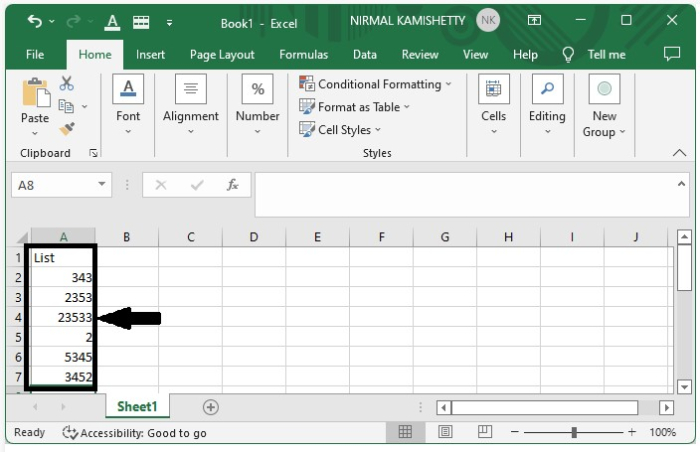To add pad leading zeros, click on the empty cell and enter the formula as =TEXT(A2,"000000") in the formula box, then click on Enter to get the first result as shown in the below image.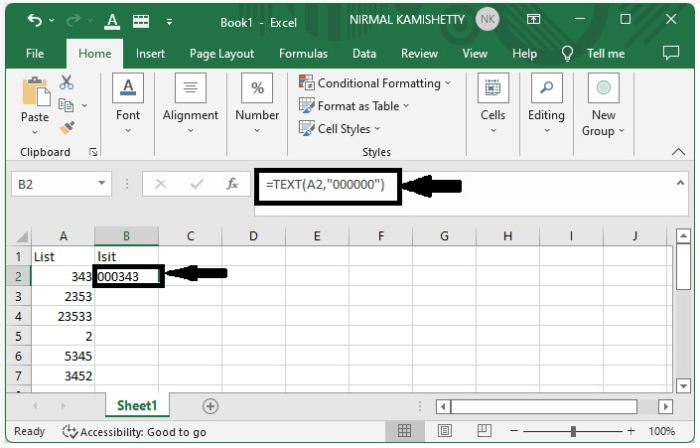Step 2

To get all the results, drag down from the cell where the first result is present until all the results are filled in.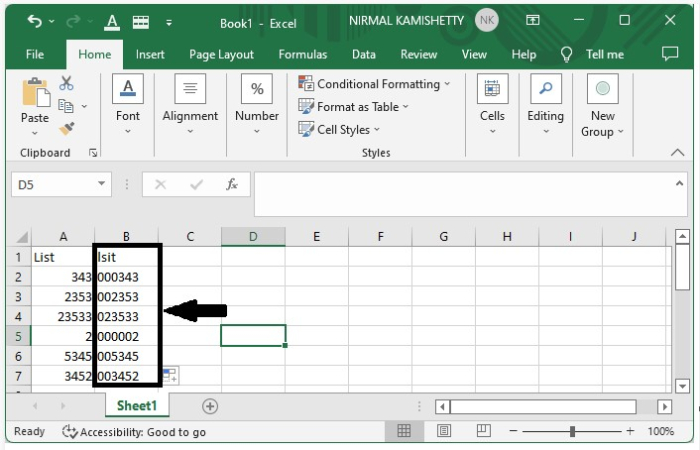Here we will use the formula to get the first result, then use the auto-fill handle to complete the task. Let's look at a simple example of how to use the CONCAT function to add leading zeros.

Step 1

Let us consider the same data that is used in the above example.

To add pad leading zeros, click on an empty cell and enter the formula =CONCAT("000",A4) in the formula box. Click on Enter to get the first result.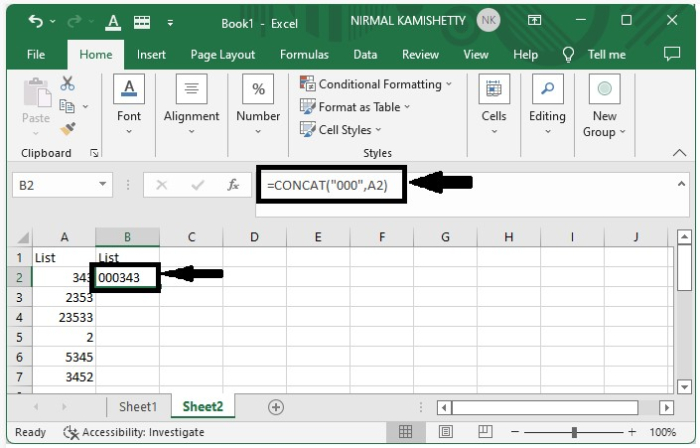Step 2

To get all the other results, drag down from the cell where the result is present, and our first result will be the same as shown in the below image.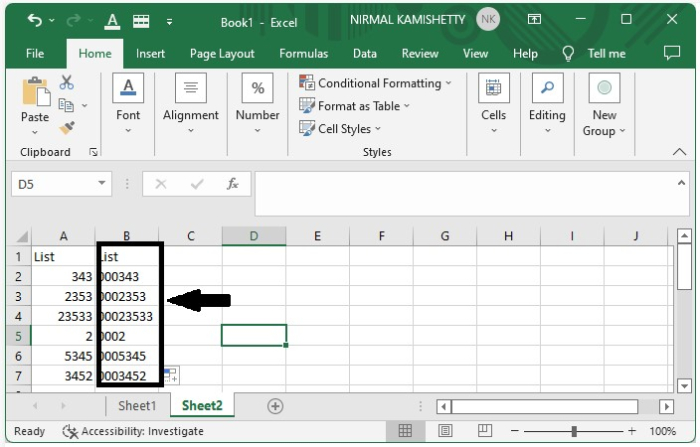Here we will use the formula to get the first result, then use the auto-fill handle to complete the task. Let's go over a simple procedure for adding pad leading zeros with the Format function.

Step 1

Let us consider the same data that we used in the above example.

To add pad leading zeros, select data, then right-click and select format cells and click on "Custom," then enter type 000000 and click on "OK" to get our result.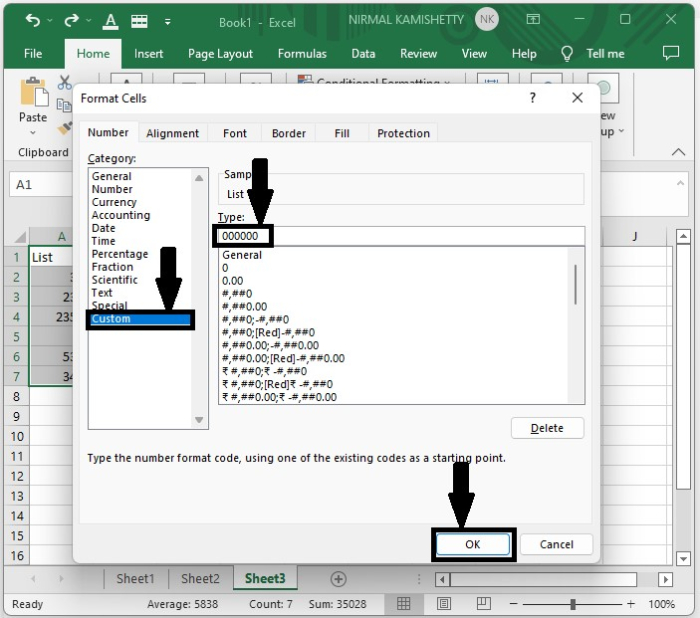The final result will look like the below image.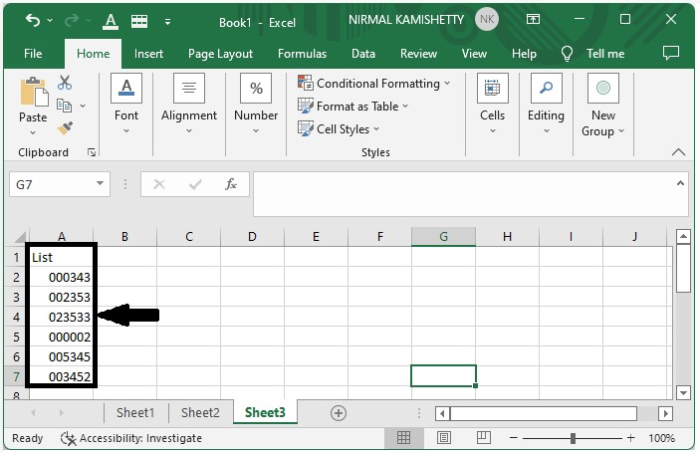## Conclusion

In this tutorial, we used a simple example to demonstrate how we can add leading zeros to the list of numbers in Excel to highlight a particular set of data.

Updated on: 09-Jan-2023

7K+ Views